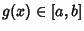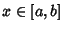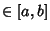## Fixed Point Theorem

Ifis a continuous functionFor All, thenhas a Fixed Point in. This can be proven by noting thatSinceis continuous, the Intermediate Value Theorem guarantees that there exists asuch thatso there must exist asuch thatso there must exist a Fixed Point.

See also Banach Fixed Point Theorem, Brouwer Fixed Point Theorem, Kakutani's Fixed Point Theorem, Lefshetz Fixed Point Formula, Lefshetz Trace Formula, Poincaré-Birkhoff Fixed Point Theorem, Schauder Fixed Point Theorem